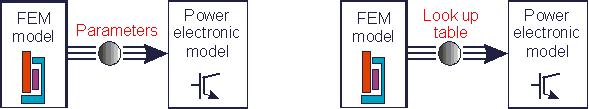• Introduction
• What is in this manual
• What is Caspoc
• User interface
• Introduction
• Starting
• Simulation
• Editing
• Viewing and printing
• Getting Started
• Basic editing
• Simulation in the time domain
• Basic User Interface Topics
• Editing
• Simulation
• Viewing
• Library
• Reports
• Project management
• Circuit and Block Diagram Components
• Introduction
• Cscript and user defined functions
• Component parameters
• Modeling Topics
• Introduction
• Power Electronics
• Semiconductors
• Electrical Machines
• Electrical drives
• Power Systems
• Mechanical Systems
• Thermal Systems
• Magnetic Circuits
• Green Energy
• Coupling to FEM
• Experimenter
• Analog hardware description language
• Embedded C code Export
• Coupling to Spice
• Small Signal Analysis
• Matlab coupling
• Tips and tricks
• Appendices

## Exporting parameters from FEM.

Depending on the application there are various parameters that can be calculated in a FEM analysis and exported to Caspoc. In the following we discuss some typical applications and what should be exported from FEM.The applications discussed below are:

• Inductance
Exporting the values of inductors or coupled inductors.
• Capacitance
Exporting the capacitance to be used in any circuit simulation
• Transformers
Exporting the primary and secondary inductance, coupling as well as the winding resistance of transformers and coupoled inductors
• Planar transformer
Winding inductance of the primary and secondary side of aPCB traces
• BLDCM Brushless motors
Back emf and torque look-up tables
• PMSM Permanent Magnet Synchronous Machines
Back emf and torque look-up tables and secondly the current dependent winding inductance.
• Induction machines
Saturating magnetizing inductance
• Wireless power transfer
Transmitter and receiver winding inductance as well as coupling as function of distance and displacement
• Heatsink
Thermal impedance from FEM translated into a Cauer model in Caspoc
• Drive line inertia and friction
Inertia of a drive shaft with multiple masses and diameters, as well as coulomb, viscous and stiction friction
• Battery impedance
Identification of charging and discharging impedance for battery efficiency and response modelling